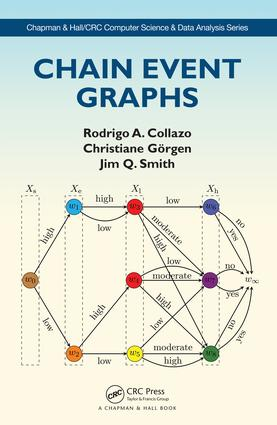Chain Event Graphs

1st Edition

CRC Press

234 pages

Purchasing Options:\$ = USD
Hardback: 9781498729604
pub: 2018-01-30
SAVE ~\$18.79
\$93.95
\$75.16
x
eBook (VitalSource) : 9781315120515
pub: 2018-01-29
from \$46.98

FREE Standard Shipping!

Description

Written by some major contributors to the development of this class of graphical models, Chain Event Graphs introduces a viable and straightforward new tool for statistical inference, model selection and learning techniques. The book extends established technologies used in the study of discrete Bayesian Networks so that they apply in a much more general setting

As the first book on Chain Event Graphs, this monograph is expected to become a landmark work on the use of event trees and coloured probability trees in statistics, and to lead to the increased use of such tree models to describe hypotheses about how events might unfold.

Features:

• introduces a new and exciting discrete graphical model based on an event tree
• focusses on illustrating inferential techniques, making its methodology accessible to a very broad audience and, most importantly, to practitioners
• illustrated by a wide range of examples, encompassing important present and future applications
• includes exercises to test comprehension and can easily be used as a course book
• introduces relevant software packages

Rodrigo A. Collazo is a methodological and computational statistician based at the Naval Systems Analysis Centre (CASNAV) in Rio de Janeiro, Brazil. Christiane Görgen is a mathematical statistician at the Max Planck Institute for Mathematics in the Sciences, Leipzig, Germany. Jim Q. Smith is a professor of statistics at the University of Warwick, UK. He has published widely in the field of statistics, AI, and decision analysis and has written two other books, most recently Bayesian Decision Analysis: Principles and Practice (Cambridge University Press 2010).

Reviews

"Statisticians Collazo, Görgen, and Smith provide a thorough introduction to the methodology of chain event graphs. The authors present background on discrete statistical modeling and the use of Bayesian inference. The chain event graph method is shown to be less restrictive than that of Bayesian networks, though it represents something of a generalization of that method. Beginning with an event tree, the chain event graph is a graphical representation that can represent a process of developing events. The authors present an array of examples to illustrate the concepts, and exercises are scattered throughout the text. Included with the book's purchase is access to software to create these models. Readers interested in this subject may also wish to consult the works of Judea Pearl, who developed Bayesian Networks and promoted the use of a probabilistic approach to the field of artificial intelligence (see, for example, Causality: Models, Reasoning, and Inference, CH, Mar'10, 47-3771)."

~CHOICE, R. L. Pour, emeritus, Emory and Henry College

Summing Up: Recommended. Upper-division undergraduates through faculty and professionals.

1.Introduction

Some motivation

Why event trees?

Using event trees to describe populations

How we have arranged the material in this book

Exercises

2.Bayesian inference using graphs

Inference on discrete statistical models

Two common sampling mass functions

Two prior-to-posterior analyses

Poisson–Gamma and Multinomial–Dirichlet

MAP model selection using Bayes Factors

Statistical models and structural hypotheses

An example of competing models

The parametric statistical model

Discrete Bayesian networks

Factorisations of probability mass functions

The d-separation theorem assumptions

Estimating probabilities in a BN

Propagating probabilities in a BN

Concluding remarks

Exercises

3.The Chain Event Graph

Models represented by tree graphs

Probability trees

Staged trees

The semantics of the Chain Event Graph

Comparison of stratified CEGs with

Examples of CEG semantics

The saturated CEG

The simple CEG

The square-free CEG

Some related structures

Exercises

4.Reasoning with a CEG

Encoding qualitative belief structures with CEGs

Vertex- and edge-centred events

Intrinsic events

Conditioning in CEGs

Vertex-random variables, cuts and independence

CEG statistical models

Parametrised subsets of the probability simplex

The swap operator

The resize operator

The class of all statistically equivalent staged trees

Exercises

5.Estimation and propagation on a given CEG

Estimating a given CEG

A conjugate analysis

How to specify a prior for a given CEG

Example: learning liver and kidney disorders

When sampling is not random

Propagating information on trees and CEGs

Propagation when probabilities are known

Example: propagation for liver and kidney disorders

Propagation when probabilities are estimated

Exercises

6.Model selection for CEGs

Calibrated priors over classes of CEGs

Log-posterior Bayes Factor (lpBF) scores

CEG greedy and dynamic programming

Greedy SCEG search using AHC

SCEG exhaustive search using

Technical advances for SCEG model selection

DP and AHC using a block ordering

A pairwise moment non-local prior (pm-NLP)

Exercises

7.How to model with a CEG: a real-world application

Previous studies and domain knowledge

Searching the CHDS dataset with a variable order

Searching the CHDS dataset with a block ordering

Searching the CHDS dataset without a variable ordering

Issues associated with model selection

Exhaustive CEG model search

Searching the CHDS dataset using NLPs

Setting a prior probability distribution

8.Causal inference using CEGs

Bayesian networks and causation

Extending a BN to a causal BN

Problems of describing causal hypotheses using a BN

Defining a do-operation for CEGs

Composite manipulations

Example: student housing situation

Some special manipulations of CEGs

Causal CEGs

When a CEG can legitimately be called ‘causal

Example: manipulations of the CHDS

Backdoor theorems

Causal discovery algorithms for CEGs

Exercises

Bibliography